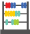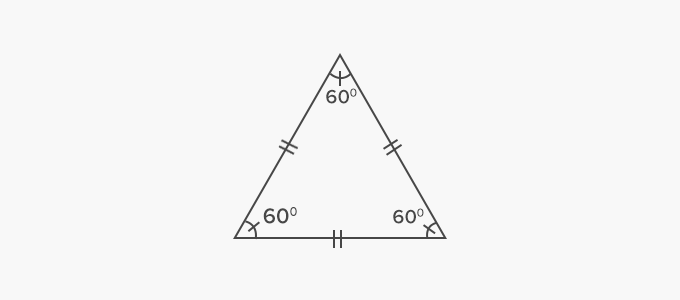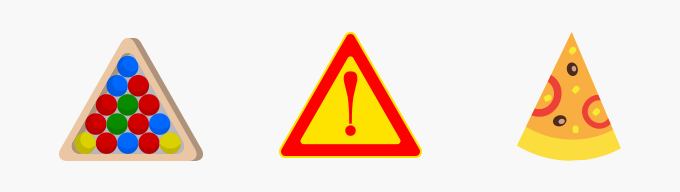# Equilateral Triangle - Definition with Examples

The Complete K-5 Math Learning Program Built for Your Child

• 30 Million Kids

Loved by kids and parent worldwide

• 50,000 Schools

Trusted by teachers across schools

• Comprehensive Curriculum

Aligned to Common Core

##Let's learn!

What is an equilateral triangle?
In geometry, an equilateral triangle is a triangle whose all three sides are of equal length.The sides of an equilateral triangle are congruent.We can find the shape of a triangle in a rack in billiards, a road side sign board and a slice of pizza, around us.Fun Facts All angles of an equilateral triangle measure 60 degrees (60°).

##Let's sing!

Sides of an equilateral triangle
Measure the same,
Like in a road side sign board
Or a rack of billiards game!

##Let's do it!

Instead of handing out math geometry worksheets on triangles, ask children to observe/spot things around them in which they can see equilateral triangles.
You can also ask them to measure sides of various triangles they draw and find out if it is an equilateral triangle or not.

##Related math vocabulary

Won Numerous Awards & Honors